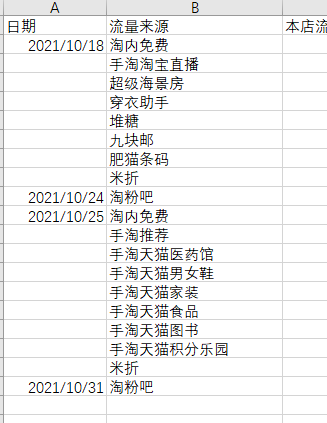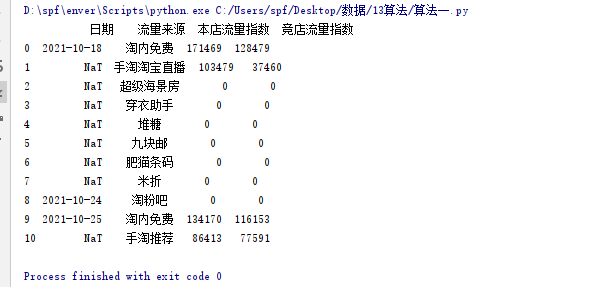###### 单雄信
2021-11-04 13:30浏览 29

# pandas删除查找索引指定行数``````import pandas as pd
import time
start = time.clock()
df1 = df['日期'].astype('str')
df 1= list(df1)
aa=df.index('2021-10-25')+2 //索引
df1 = df.drop(index=range(aa,19,1),inplace=True)
print(df1)

``````